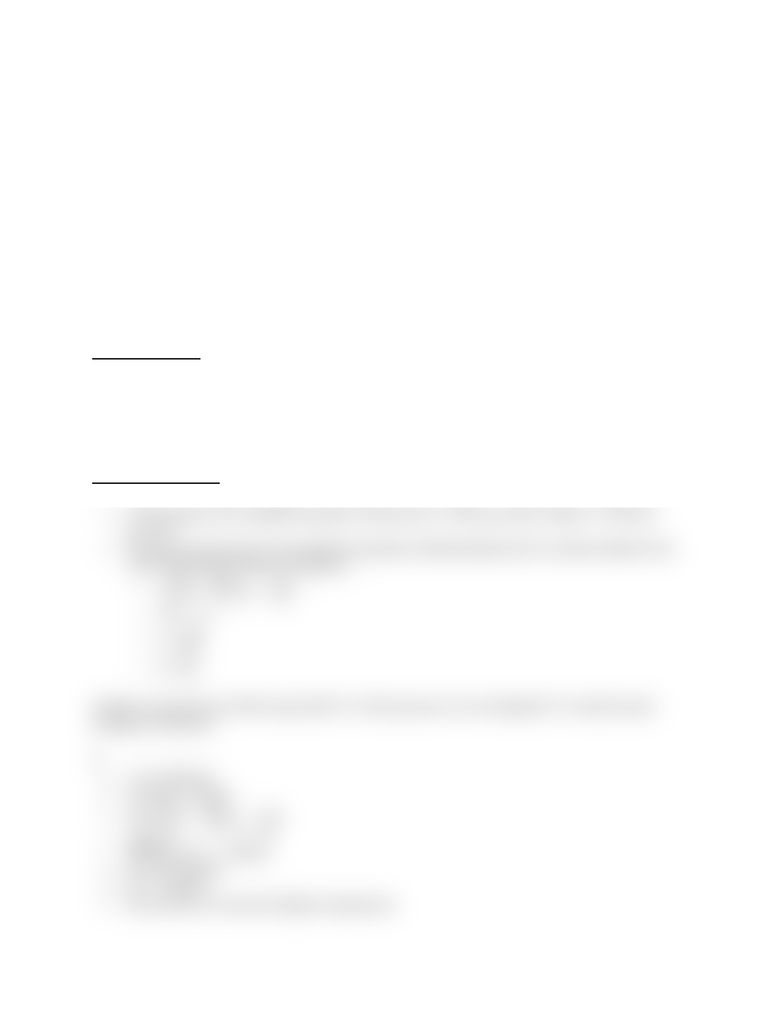# CHEM 1310 Lecture Notes - Stoichiometry, Rate Equation, Joule

79 views2 pages
School
Department
Course
ProfessorG reaction = products - reactants
S - what can you conclude about the sign and magnitude?
G = H - TS
o H = 34.5
o T = 450
o G = -1
o -1 = 34.5 kJ -450S
o - 35500 = -450S
o S = 78.9
Q.Calculate standard reaction entropy at 298 K for:
o 2CH3CHO + O2 2CH3COOH
A. Sᵒ = 159.8(2) - 263.8(2) - 205.2
Gibb's Free Energy
Is a state function (constant)
Gives the amount of useable energy in the universe
May be calculated using G = H - TS
G < 0 means reaction is spontaneous
The value for a reaction may be manipulated using the same methods as H and S
Hᵒ and Temperature
Clausius-Clapeyron often used to relate Hᵒ and Sᵒ to temperature and pressure
Is the equation of a straight line graph on lnP(y-axis) vs. 1/T(x-axis with m=slope= Hᵒ/R and
b=Sᵒ/R
There are several ways to rearrange this equation mathematically and it is closely related to the
van't Hoff and the Arrhenius equations
o 
 󰀫


o M =
o X = 󰀫
o B = 
Q.What is the pressure of H2O vapor @ 64 ᵒC, if the pressure is 22.4 mmHg @ 23 ᵒC and the molar
enthalpy is 44 kJ/mol
A.
H = 44 kJ/mol
Constant = 8.3145
Use ln 
 






P1 = 22.4 mmHg
P2 = ? mmHg
Hint: pick set 2 to have the higher temperature
Unlock document

This preview shows half of the first page of the document.
Unlock all 2 pages and 3 million more documents.

# Get access

\$10 USD/m
Billed \$120 USD annually
Homework Help
Class Notes
Textbook Notes